# Constructing a 60 Degree Angle and an Equilateral Triangle

Compass and straight edge constructions are of interest to mathematicians, not only in the field of geometry, but also in algebra. For thousands of years, beginning with the Ancient Babylonians, mathematicians were interested in the problem of "squaring the circle" (drawing a square with the same area as a circle) using a straight edge and compass. This problem is equivalent to finding the area of a circle. It turns out that this is impossible, but no-one managed to prove this until 1882!

However, it is possible to construct a 60 degree angle and an equilateral triangle using only a straight edge and compass. No cheating and using a protractor or a set square! For this construction, you will need a straight edge (ruler - but you won't be measuring anything), pair of compasses, a pencil and paper. I have drawn the pictures using the robocompass app. It's fun to play with, and you can use it to do all sorts of geometric constructions. There's a little bit of coding to learn, but a list of instructions is provided. Once you've written your little program, you can get out the popcorn, sit back and watch the construction. Be warned that, when you are using it to draw arcs, the robocompass compass point may appear to be a little away from the centre, but the drawing is actually accurate.

Both constructions involve building a 60 degree angle. You can stop after this if you only want a 60 degree angle. There's just one extra step required to turn your 60 degree angle into an equilateral triangle.

## Constructing the 60 Degree Angle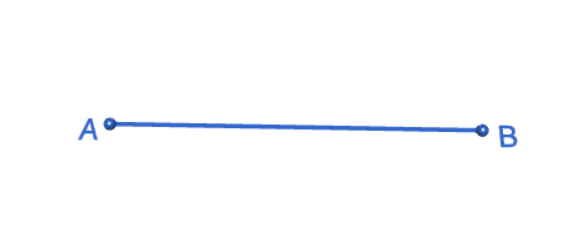Step 1: Start out by drawing the line segment $AB$ that you want to base your angle on. The point $A$ will be the vertex of your angle. Please don't be like Sam! Use a ruler to draw this.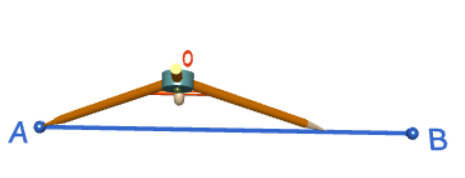Step 2: Place the tip of your pair of compasses on point $A$, and open them out to a comfortable size (it doesn't really matter, but don't make it too small, or you won't have room to move). You are going to draw two arcs of this radius, so make sure you keep it fixed. Draw an arc from almost vertically above the point $A$ down to pass through the line segment. The arc is centred at point $A$, so make sure your compass tip is exactly on point $A$. The picture on the right shows what your construction should look like when you've finished this step.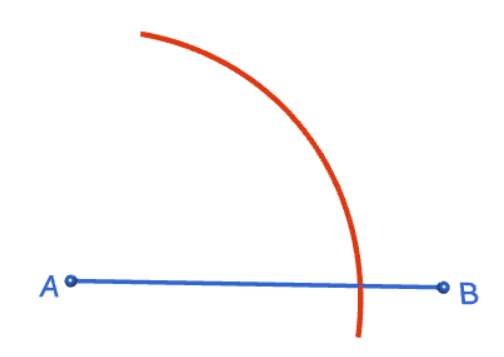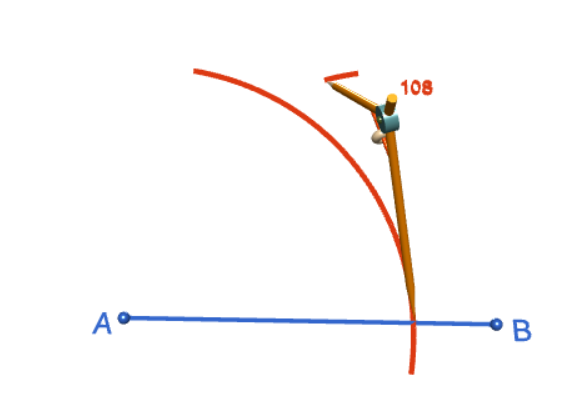Step 3: Keeping your compasses at the same radius as in the preceding step, place the tip of your pair of compasses at the point where your arc from step 2 cuts through the line segment. Draw an arc that cuts your first arc at a point $C$. The arc should be centred at the point of intersection of the first arc and the line segment, so make sure your compass tip is exactly on this point of intersection. As shown in the picture, your arc needs to intersect the arc you drew in step 2. The picture on the right shows what your construction should look like when you've finished this step.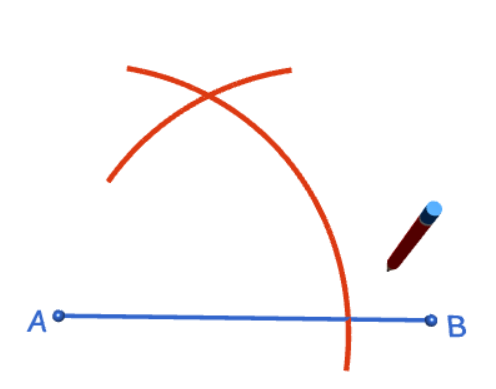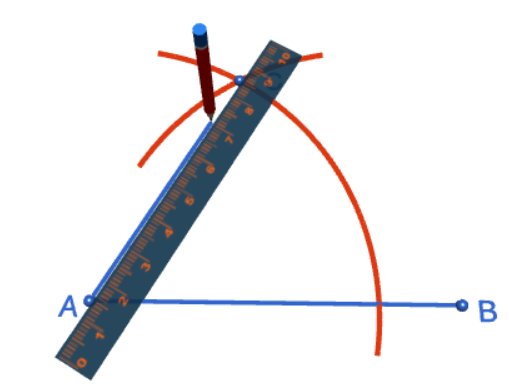Step 4: Use your ruler to draw a line segment joining points $A$ and $C$. This is the second arm of your $60^\circ$ angle. The picture on the right shows the completed construction. You can check the angle using a protractor, if you like.## Finishing the Triangle

We only need one more step to create a beautiful equilateral triangle.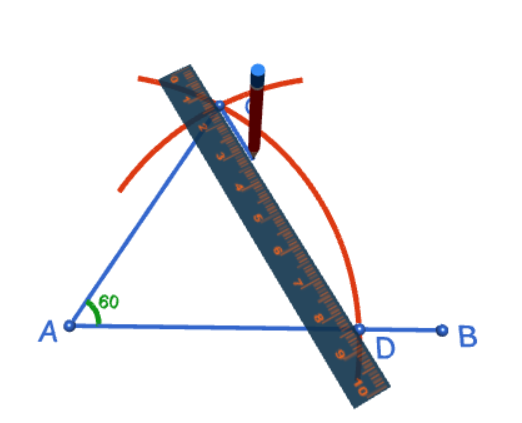Step 5: Label the point where the arc from step 2 cuts line segment $AB$ with $D$. Use your ruler to draw a line segment joining points $D$ and $C$. This is the third side of your equilateral triangle. The picture on the right shows the completed equilateral triangle. You can check that all the angles are $60^\circ$ using a protractor, if you like.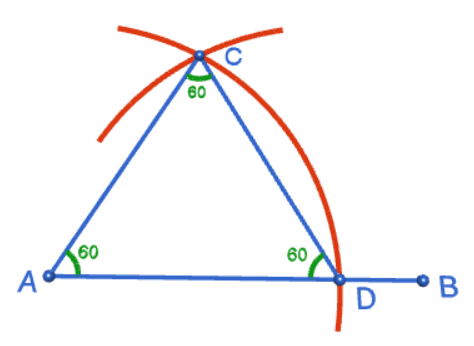### Description

This tutorial will expose you to Rulers and how to use them. We will also have a close look as set square and compass constructions. Your feedback is important to us, if you like any other topic covered under this tutorial, please do let us know.

### Audience

Year 10 or higher

### Learning Objectives

Ruler and compass constructions and more

Author: Subject Coach
You must be logged in as Student to ask a Question.